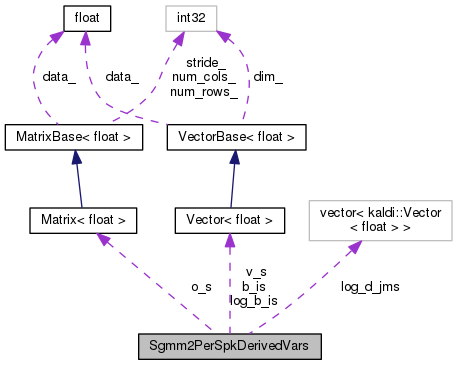Sgmm2PerSpkDerivedVars Class Reference

`#include <am-sgmm2.h>`

Collaboration diagram for Sgmm2PerSpkDerivedVars:[legend]

## Public Member Functions

void Clear ()

bool Empty ()

const Vector< BaseFloat > & GetSpeakerVector ()

void SetSpeakerVector (const Vector< BaseFloat > &v_s_in)

## Protected Attributes

Vector< BaseFloatv_s
Speaker adaptation vector v_^{(s)}. Dim is [T]. More...

Matrix< BaseFloato_s
Per-speaker offsets o_{i}. Dimension is [I][D]. More...

Vector< BaseFloatb_is

Vector< BaseFloatlog_b_is
< [SSGMM]: Eq. (22) in techreport, b_i^{(s)} = (^T ^{(s)}) More...

std::vector< Vector< BaseFloat > > log_d_jms
< [SSGMM] log of the above (more efficient to store both). More...

## Friends

class AmSgmm2

class MleAmSgmm2Accs

## Detailed Description

Definition at line 165 of file am-sgmm2.h.

## ◆ Clear()

 void Clear ( )
inline

Definition at line 168 of file am-sgmm2.h.

Referenced by AmSgmm2::ComputePerSpkDerivedVars(), and main().

168  {
169  v_s.Resize(0);
170  o_s.Resize(0, 0);
171  b_is.Resize(0);
172  log_b_is.Resize(0);
173  log_d_jms.resize(0);
174  }
Vector< BaseFloat > v_s
Speaker adaptation vector v_^{(s)}. Dim is [T].
Definition: am-sgmm2.h:187
Vector< BaseFloat > b_is
Definition: am-sgmm2.h:189
std::vector< Vector< BaseFloat > > log_d_jms
< [SSGMM] log of the above (more efficient to store both).
Definition: am-sgmm2.h:191
void Resize(const MatrixIndexT r, const MatrixIndexT c, MatrixResizeType resize_type=kSetZero, MatrixStrideType stride_type=kDefaultStride)
Sets matrix to a specified size (zero is OK as long as both r and c are zero).
Vector< BaseFloat > log_b_is
< [SSGMM]: Eq. (22) in techreport, b_i^{(s)} = (^T ^{(s)})
Definition: am-sgmm2.h:190
Matrix< BaseFloat > o_s
Per-speaker offsets o_{i}. Dimension is [I][D].
Definition: am-sgmm2.h:188

## ◆ Empty()

 bool Empty ( )
inline

Definition at line 175 of file am-sgmm2.h.

Referenced by main().

175 { return v_s.Dim() == 0; }
Vector< BaseFloat > v_s
Speaker adaptation vector v_^{(s)}. Dim is [T].
Definition: am-sgmm2.h:187

## ◆ GetSpeakerVector()

 const Vector& GetSpeakerVector ( )
inline

Definition at line 178 of file am-sgmm2.h.

Referenced by main().

178 { return v_s; }
Vector< BaseFloat > v_s
Speaker adaptation vector v_^{(s)}. Dim is [T].
Definition: am-sgmm2.h:187

## ◆ SetSpeakerVector()

 void SetSpeakerVector ( const Vector< BaseFloat > & v_s_in )
inline

Definition at line 180 of file am-sgmm2.h.

References VectorBase< Real >::Dim().

Referenced by main(), and kaldi::ProcessUtterance().

180  {
181  v_s.Resize(v_s_in.Dim());
182  v_s.CopyFromVec(v_s_in);
183  }
Vector< BaseFloat > v_s
Speaker adaptation vector v_^{(s)}. Dim is [T].
Definition: am-sgmm2.h:187

## ◆ AmSgmm2

 friend class AmSgmm2
friend

Definition at line 185 of file am-sgmm2.h.

## ◆ MleAmSgmm2Accs

 friend class MleAmSgmm2Accs
friend

Definition at line 186 of file am-sgmm2.h.

## ◆ b_is

 Vector b_is
protected

Definition at line 189 of file am-sgmm2.h.

## ◆ log_b_is

 Vector log_b_is
protected

< [SSGMM]: Eq. (22) in techreport, b_i^{(s)} = (^T ^{(s)})

Definition at line 190 of file am-sgmm2.h.

Referenced by AmSgmm2::ComputePerFrameVars(), and AmSgmm2::ComputePerSpkDerivedVars().

## ◆ log_d_jms

 std::vector > log_d_jms
protected

< [SSGMM] log of the above (more efficient to store both).

[SSGMM] normalizers per-speaker and per-substate; indexed [j1][m].

Definition at line 191 of file am-sgmm2.h.

## ◆ o_s

 Matrix o_s
protected

Per-speaker offsets o_{i}. Dimension is [I][D].

Definition at line 188 of file am-sgmm2.h.

## ◆ v_s

 Vector v_s
protected

Speaker adaptation vector v_^{(s)}. Dim is [T].

Definition at line 187 of file am-sgmm2.h.

The documentation for this class was generated from the following file: